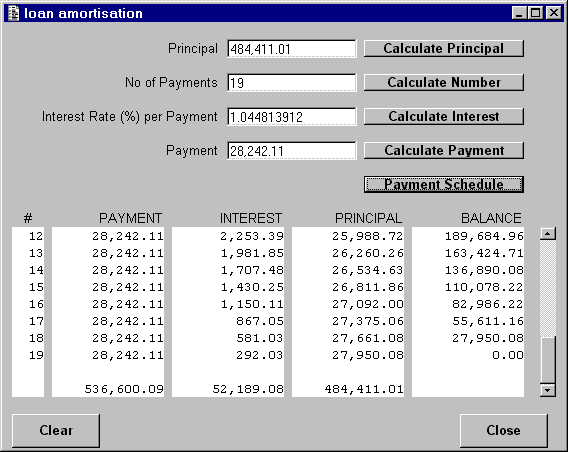# Tony Marston - 23rd July 2000

I have seen several requests in the newsgroup for help on doing loan calculations, and as I have already written such routines in COBOL and Visual Basic I thought I would try and produce one in UNIFACE.

NOTE: I have subsequently produced a version in PHP which is described here and which can be run online here.

The one featured in the sample code is for a loan with end-of-period payment. The four elements in each equation are:

 ```PV = Principal Value PMT = Payment Amount PAYNO = Number of Payments INT = Interest Rate per Payment ```

The sample program allows any three of these values to be entered and it will calculate the fourth. It also has a button which will produce a schedule of payments showing how each payment is split between interest and principal. Here is a screen shot of the sample program:The formulae were obtained from the instruction manual for a Casio BF-100 calculator that I bought in 1984 when working on a Lease Accounting System.

 ``` (1 + INT)PAYNO - 1 PV = PMT * ------------------- INT * (1 + INT)PAYNO ``` ``` log(1 - INT * PV/PMT) PAYNO = --------------------- log(1 + INT) ``` ``` INT * (1 + INT)PAYNO PMT = PV * ------------------- (1 + INT)PAYNO - 1 ``` ```INT = Approximation formulas by Newton's Law ```

For those of you who don't understand the meaning of 'approximation formulas' it roughly means taking a guess at an interest rate and calculating one of the other known values. If the calculated value does not match the known value then keep adjusting the guessed interest rate until they do match. The method of adjusting the guessed interest rate is known as 'the binary chop technique', but you will have to look at my sample code to see what that means.

If anybody wishes examples using other types of calculation please let me know and I'll see what I can do.

Tony Marston
23rd July 2000

mailto:tony@tonymarston.net
mailto:TonyMarston@hotmail.com
http://www.tonymarston.net